# HomeworkHelpOnline.net Blog

We have accumulated huge amount of experience and we are sharing it with you in the form of tutorials for your best learning experience.These pages are safe to use and can save you a lot of money and time in your pursuit of a university degree.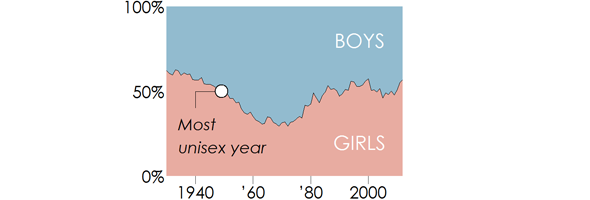### Top Unisex Baby Names of Last Century: R Studio Visualization

In this post, we combine data-wrangling and visualization skills to create a professional-looking data visualization using the `ggplot2` package in R. Most popular unisex baby names are presented nicely in an R graph.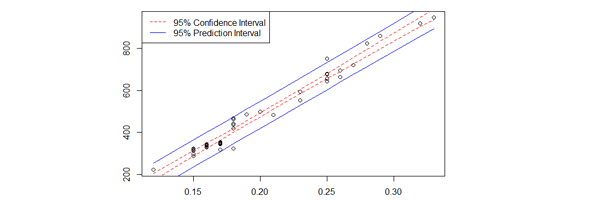### ANOVA, Linear and Logistic Regression, Chi-Square Test

Six problems exploring ANOVA, Linear and Logistic Regression, Chi-Square Test. And corresponding solutions in R Studio by our experts.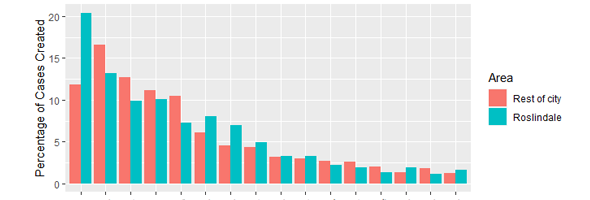### Dataset "311 Service Requests" Analysis Using tidyverse R Library

It is a popular dataset used to analyze service requests of various cities (Pitsburg, New York, etc.). This time we analyze Roslindale city in R.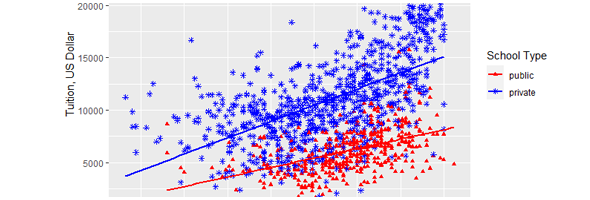### Compare R Studio ggplot2 Graphs: qplot() vs ggplot()

R Studio ggplot2 library contains functions qplot() and ggplot(). What is the result of these functions? Example solution explains the differences.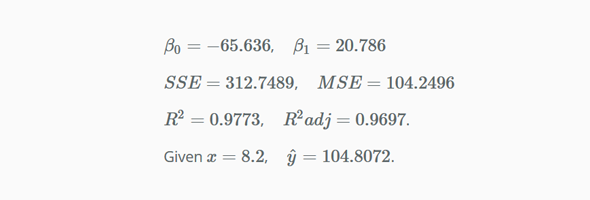### Linear Regression in Matrix Form - R Studio

The following exercises aim to compare simple linear regression results computed in matrix form with the built in R function lm().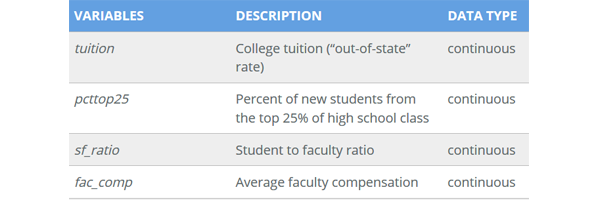### Linear Regression Analysis of College Tuition in R

The following exercises aim to use linear regression tools in R to investigate whether a linear relationship exists between tuition and other variables.

Page 1 of 3